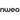# How to Add Fractions: 3 easy steps [+5 Awesome Activities]

Laney Kennedy

All Posts
Your students might know their way around a numerator and denominator, but are they ready for what’s next? Suddenly, it’s time to learn how to add fractions -- and your class is confused.Feeling intimidated? You’re not alone. Adding fractions may seem daunting, but it doesn’t need to be.We’ve put together a guide to help you successfully teach your students how to add fractions, covering:

## Why do students struggle with fractions?

Fractions -- especially fraction operations -- are a tricky subject for most students. Trouble with fractions can reduce confidence in math and lead to math anxiety, if students don’t receive enough support in the subject.Fractions are a struggle for a few reasons. Research has found the biggest issues are:

### 1. Understanding what the numbers mean

Before fractions, students are used to working with whole numbers: basic numbers that represent whole amounts. Fractions introduce students to rational numbers, which come with a whole new set of rules and patterns. The meaning behind fractions is confusing when you compare them to whole numbers. Whole numbers are only expressed one way, while fractions can be expressed in many ways and still represent the same amount. For instance, there’s only one way to represent the number three, but ²⁄₄ represents the same amount as ½, 0.5 and 50%. As a student, this is hard to wrap your head around.

### 2. Different operations for whole numbers and fractions

The methods you use to add, subtract, multiply and divide whole numbers are different than doing the same operations for fractions. Rules become much more unpredictable and confusing. Many students and  teachers have a limited understanding of how or why these methods are used. Fractions are harder to represent with visuals or manipulatives, and the rules for adding them are more difficult to understand. Learning how to multiply and divide fractions can add even more confusion, as students must remember the differences between these operations. This is a big adjustment for students who are already comfortable with whole number arithmetic.## Types of fractions

Students must first understand the difference between each type of fraction to successfully add them.First, let’s start with the basic components of a fraction. A fraction represents parts of a whole. The numerator (the top number) illustrates the number of parts you have. The denominator (the bottom number) shows the total number of parts the whole is divided into.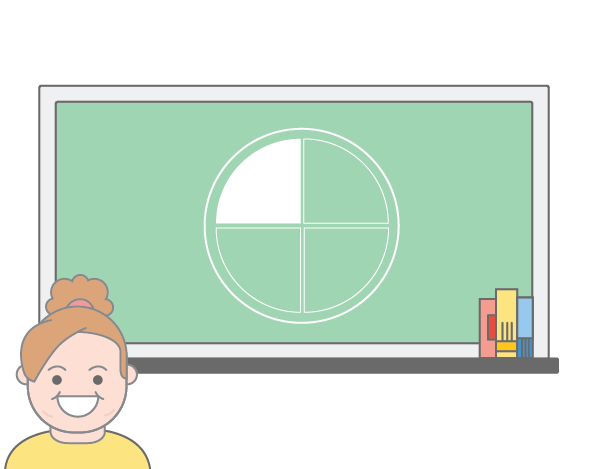In the illustration above, our circle is divided into four parts. This means four is our denominator. Of those four parts, one is highlighted. This means one is our numerator. So, our fraction is ¼ or one quarter.There are three general categories of fractions: Proper, improper and mixed.
 Type Meaning Example Proper fraction The numerator is less than the denominator ¾ (three quarters) Improper fraction The numerator is greater than the denominator ⁷⁄₄ (seven quarters) Mixed fraction A whole number and a proper fraction combined 1 ¾ (one and three quarters)
In addition to these, fraction equations will be split into two distinct categories: those with like fractions and those with unlike fractions.
 Type Meaning Example Like fractions Fractions with the same denominator ¼ and ¾ Unlike fractions Fractions with different denominators ¼ and ⅜
A base knowledge of these types will help students understand what to do when faced with a question about adding fractions.Now that you’re familiar with each type of fraction, you can get adding! Teach your students the three-step formula below to confidently tackle fraction addition equations.

## 3 Easy steps for adding fractions

It may seem scary at first, but adding fractions can be easy. All you need to do is follow three simple steps:
• Step 1: Find a common denominator
• Step 2: Add the numerators (and keep the denominator)
• Step 3: Simplify the fraction
Let’s look at each step in a bit more detail.

### Step 1: Find a common denominator

If your two denominators are already the same, you’re adding fractions with like denominators. Fantastic! This means you can skip to step two.If your denominators are different, you’re adding fractions with unlike denominators. When adding unlike fractions, you need to find a common denominator so you can add the two fractions together.Check out the video below to understand why we need a common denominator to add fractions.You can find the common denominator using equivalent fractions: fractions that have the same value. For instance, ²⁄₄, ³⁄₆ and ⁴⁄₈ are equivalent fractions because they can all be reduced to ½. There are two main methods for finding the common denominator.

#### 1) The common denominator method

In this method, you’ll multiply the top and bottom of each fraction by the denominator of the other. For example, consider the following equation:

# ⅓ + ⅙

Our fractions have two different denominators: three and six. We need to multiply the numerator and denominator in ⅓ by six, then multiply the numerator and denominator in ⅙  by three. When we do this, our new fractions become ⁶⁄₁₈ and ³⁄₁₈.The two new fractions have the same denominator, so now we can add them!

#### 2) The least common denominator method

This method involves finding the smallest of all common denominators, then multiplying your original fractions to get that denominator.To find the least common denominator, list all the multiples of the number and find the smallest number that’s the same among them.For example, using the same equation as before -- ⅓ + ⅙ -- you can put together a table to determine the smallest common multiple.
 Multiplied by: Denominator 1 Denominator 2 x1 3 6 x2 6 12 x3 9 18 x4 12 24 x5 15 30 x6 18 36
As you can see from our table, the smallest multiple that’s the same is six.So, for ⅓ , both the numerator and denominator must be multiplied by two to get ²⁄₆. For ⅙, the numbers must be multiplied by one, so the fraction stays the same. Once again, our fractions are ready to be added!

### Step 2: Add the numerators (and keep the denominator)

This step is rather straightforward. Add your numerators together so the sum becomes the new numerator, while the denominator stays the same.  Let’s use our previous example: ⅓ + ⅙Using our new equation from the common denominator method -- ⁶⁄₁₈ + ³⁄₁₈ -- we need to add six and three together. The denominator will still be eighteen.Six plus three is nine, so our answer is ⁹⁄₁₈.

### Step 3: Simplify the fraction

If your fraction contains high numbers, you may need to simplify it. Simplifying involves finding the smallest equivalent fraction possible. In our previous equation, our answer was ⁹⁄₁₈. This number seems a bit large, so we'll see if we can simplify it to an easier number. To simplify a fraction, you need a common factor: a number that will divide into both numbers evenly. For example, two is a common factor of four and six, because both numbers can be divided by two. The two easiest methods for simplifying a fraction are:

#### 1) Trial and error

For this method, just keep dividing the numerator and denominator by small numbers. Start with two, then three, then four -- and so on until you get the smallest possible answer.With our answer of ⁹⁄₁₈, we can keep trying to divide by small numbers until we find one that works. Can both nine and eighteen be divided by two? No. We can’t divide nine by two evenly. Ok, let’s try another number. Can both nine and eighteen be divided by three? Yes! When we divide both by three, our fraction becomes ³⁄₆.Now that we have a simpler answer, it’s time to see if we can we simplify even further. Three and six can both be divided by three again, so our final answer is ½.

#### 2) Find the Greatest Common Factor (GCF)

The GCF is the highest number that divides evenly into two or more numbers. This method is similar to finding the least common denominator -- you’ll find the answer by listing all possible factors. Using our previous example of ⁹⁄₁₈, we’ll find and list all the factors of each number, starting from one. Once you've listed all of the factors of that number, all you have to do is find the largest number repeated in both lists.A handy table helps for this, too.
 Factors of 9 Factors of 18 1 1 3 2 9 3 6 9 18
We’ll use our table to find the largest number common to both numbers. In this case, the greatest common factor for nine and eighteen is nine. Now we can divide both numbers by nine to get our reduced fraction: ½.When you put all three steps of adding fractions together, it looks like this: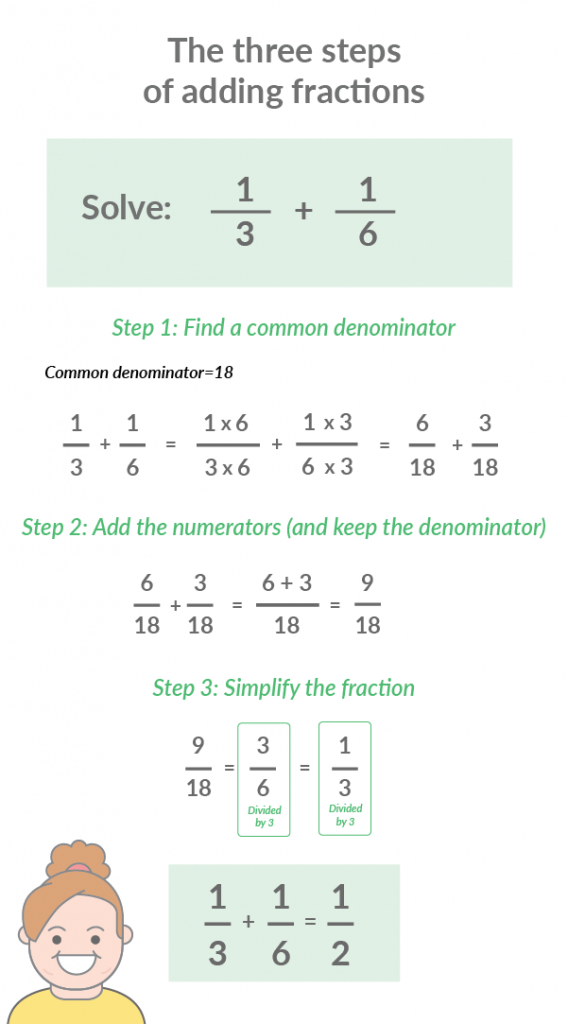The above steps work great for proper and improper fractions, but what about adding fractions with whole numbers?Adding mixed fractions is actually quite simple: just convert it to an improper fraction and you’re ready to start adding!Every mixed fraction can be made into an improper fraction. For instance, 1 ¾ is the same thing as ⁷⁄₄.Image source: Central Bucks School DistrictThere are three steps for converting mixed fractions to improper fractions:

### 1. Multiply the whole number by the denominator

Let’s use 1 ¾. If we multiply our whole number (one) by our denominator (four), we get four.

### 2. Add that number to the numerator

Our new number (four) plus our numerator (three) is seven.

### 3. Write your new numerator over the original denominator

Our new numerator (seven) over our original denominator (four) equals ⁷⁄₄.Now you can add the fraction!## The importance of adding fractions

As a teacher, you’re probably quite familiar with the age-old question students ask: “why am I even doing this?” In this context, it’s certainly a valid question. Why is adding fractions so important to learn?For starters, there are many real-life applications of this arithmetic. On many occasions, you’ll need to find the total number of parts of a whole when they’re combined. Here are a few potential examples of adding fractions in real life:
1. Exercising: If you run a ¼ mile on Monday and ¾ mile on Tuesday, how far did you run over both days?
2. Time-management: If you work 8 ½ hours on Monday and 6 ¾ hours on Tuesday, how many hours did you work over both days?
3. Cooking/baking: If you add ½ cup of milk chocolate chips and ⅓ cup of white chocolate chips to your cookie dough, what’s the total amount of chocolate chips in your recipe?
If that wasn’t enough, proficiency with fraction operations is actually quite important for learning more advanced math and science -- eventually leading to success in many academic or career paths. Limited knowledge in fraction operations can lead to weaker skills in later math and science. One study found that in the United States and the United Kingdom, elementary students’ fraction knowledge could predict general math abilities in high school. The survey of workplace Skills, Technology, and Management Practices (STAMP) found that 68% of employed people 18 or older used fractions in their daily work. This means a significant number of adults in the United States require a solid base knowledge of fractions and their operations. Learning these skills as early as possible is key for success in many workplaces.

## 5 Engaging activities for adding fractions

Now that you know what to teach your students about adding fractions, let’s focus on the how. Get inspired by these five engaging activity ideas to supplement your adding fractions lessons.

### 1) Prodigy

https://www.instagram.com/p/BqXXydbFTiH/?utm_source=ig_web_button_share_sheet Prodigy is a free, curriculum-aligned learning platform with over 1,500 skills for kids to practice math. You  can use it to target all kinds of fractions skills, from basic understanding to more complicated operations like addition.Prodigy takes players through an exciting adventure, where they answer math questions to "battle" other characters. Students are so engaged with the game, they'll actually want to keep playing -- and practicing more math as a result! The platform is a great tool for lesson supplementation, homework assignments and more. It can also help you differentiate instruction and target specific trouble spots, helping each student succeed at their own pace.
"Our last test was on Fractions, and that was the first time that I had really made sure every day on Prodigy they were practicing those specific skills, and the test results were very reflective of the additional practice they'd received!"Justine Hill 3rd Grade Teacher East Syracuse-Minoa Central Schools### |

Promote some healthy competition in your classroom with an engaging board game where players “bump” each other by adding fractions to claim a spot on the board. You can find lots of bump games for various subjects. In this adding fractions edition, players must roll dice to find a relevant equation, then place their game pieces on the fraction that corresponds with the answer. The player who gets all their game pieces on the board first is the winner!

### 4) Word problems

Word problems for fraction equations provide real-life examples of the questions students are answering, helping them understand the purpose of such questions.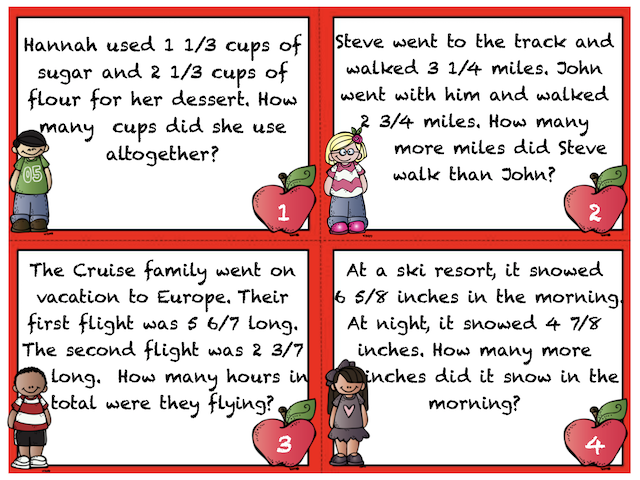Image source: Teachers Pay TeachersWord problem cards and worksheets are a great way to provide these questions. If you want your class more involved, you can use manipulatives or even the students themselves. For example, “if three people are wearing green and two are wearing blue, what’s the fraction of people wearing green or blue in the class?”

### 4) Equation architects

This activity involves students drawing or building equations to visualize what adding fractions looks like.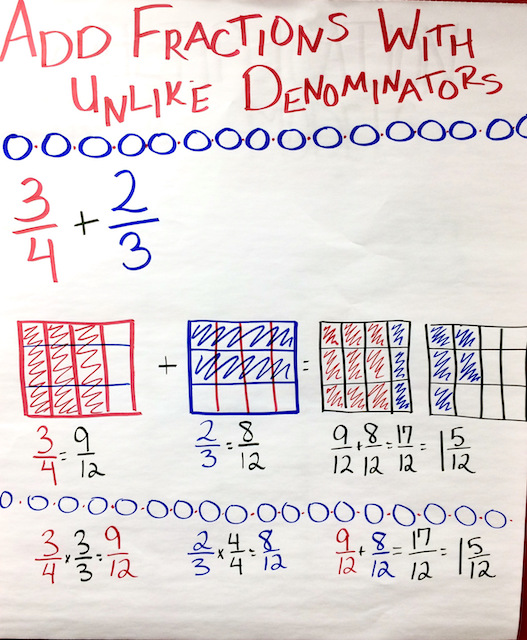Image source: Desert Designed Get your students to draw out equations or use manipulatives for a better idea of what it really means to add fractions. Fraction bars or a fraction dial are both great options to make this abstract concept more digestible and concrete. |

### 5) Math mates

This active game gets students out of their seats, collaborating with classmates and practicing math... all at once!https://www.instagram.com/p/Btmh25ulWnX/?utm_source=ig_web_button_share_sheet Each student will have a different fraction. Players go around the room finding partners and working together to add their fractions. This game is great for practicing skills learned in class and encouraging teamwork.

## Final thoughts on adding fractions

Moving from basic fraction skills to addition is certainly intimidating, but adding fractions can be made easy using the three simple steps above. Use the information in this guide to conquer your next math lesson and make adding fractions a breeze for your students. Next up: subtracting, multiplying and dividing. Oh my!>>Create or log in to your teacher account on Prodigy – a free, game-based learning platform for math that’s easy to use for educators and students alike. Aligned with curricula across the English-speaking world, it’s loved by more than a million teachers and 50 million students.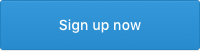•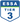•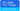•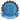•